## Series Impedance Voltmeter:

For power frequency a.c. measurements the Series Impedance Voltmeter may be a pure resistance or a reactance. Since resistances involve power losses, often a capacitor is preferred as a series reactance. Moreover, for high resistances, the variation of resistance with temperature is a problem, and the residual inductance of the resistance gives rise to an impedance different from its ohmic resistance.

High resistance units for high voltages have stray capacitances and hence a unit resistance will have an equivalent circuit as shown in Fig. 7.8. At any frequency ω of the a.c. voltage, the impedance of the resistance R is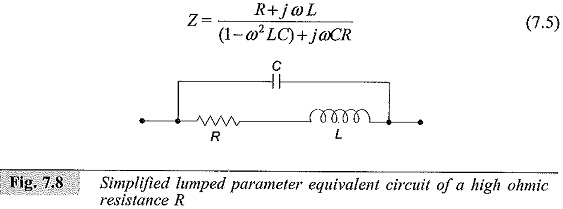where

L — Residual inductance

C — Residual capacitance

If ωL and ωC are small compared to R,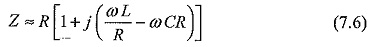and the total phase angle is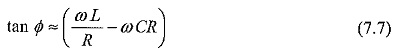This can be made zero and independent of frequency, if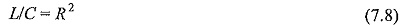For extended and large dimensioned resistors, this equivalent circuit is not valid and each elemental resistor has to be approximated with this equivalent circuit. The entire resistor unit then has to be taken as a transmission line equivalent, for calculating the effective resistance. Also, the ground or stray capacitance of each element influences the current flowing in the unit, and the indication of the meter results in an error.

The equivalent circuit of a high voltage resistor neglecting inductance and the circuit of compensated series resistor using guard and tuning resistors is shown in Fig 7.9 a and b respectively. Stray ground capacitance effects (refer Fig. 7.9b) can be removed by shielding the resistor R by a second surrounding spiral Rs, which shunts the actual resistor but does not contribute to the current through the instrument. By tuning the resistors Ra, the shielding resistor and potentials may be adjusted with respect to the actual measuring resistor so that the resulting compensation currents between the shield and the measuring resistors provide a minimum phase angle.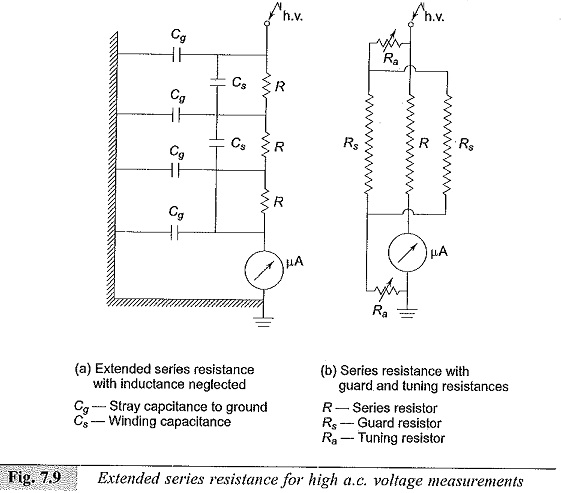where

Cg – Stray capcitance to ground

C– Winding capacitance

R – Series resistor

Rs– Guard resistor

Ra – Tuning resistor

Scroll to Top